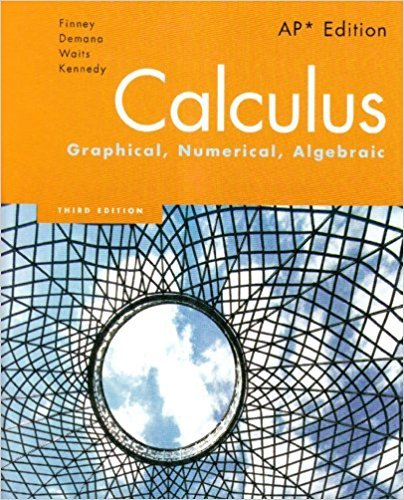×
×

# Solutions for Chapter 3: Calculus: Graphical, Numerical, Algebraic 3rd Edition## Full solutions for Calculus: Graphical, Numerical, Algebraic | 3rd Edition

ISBN: 9780132014083Solutions for Chapter 3

Solutions for Chapter 3
4 5 0 314 Reviews
14
1
##### ISBN: 9780132014083

Calculus: Graphical, Numerical, Algebraic was written by and is associated to the ISBN: 9780132014083. Chapter 3 includes 83 full step-by-step solutions. This expansive textbook survival guide covers the following chapters and their solutions. This textbook survival guide was created for the textbook: Calculus: Graphical, Numerical, Algebraic, edition: 3. Since 83 problems in chapter 3 have been answered, more than 4168 students have viewed full step-by-step solutions from this chapter.

Key Calculus Terms and definitions covered in this textbook
• Arctangent function

See Inverse tangent function.

• Common ratio

See Geometric sequence.

• Eccentricity

A nonnegative number that specifies how off-center the focus of a conic is

• End behavior

The behavior of a graph of a function as.

• Five-number summary

The minimum, first quartile, median, third quartile, and maximum of a data set.

• Geometric sequence

A sequence {an}in which an = an-1.r for every positive integer n ? 2. The nonzero number r is called the common ratio.

• Inverse cosine function

The function y = cos-1 x

• Length of a vector

See Magnitude of a vector.

• Matrix, m x n

A rectangular array of m rows and n columns of real numbers

• Nautical mile

Length of 1 minute of arc along the Earth’s equator.

• Obtuse triangle

A triangle in which one angle is greater than 90°.

• Opposite

See Additive inverse of a real number and Additive inverse of a complex number.

A function that can be written in the form ƒ(x) = ax 2 + bx + c, where a, b, and c are real numbers, and a ? 0.

• Rational zeros

Zeros of a function that are rational numbers.

• Reference triangle

For an angle ? in standard position, a reference triangle is a triangle formed by the terminal side of angle ?, the x-axis, and a perpendicular dropped from a point on the terminal side to the x-axis. The angle in a reference triangle at the origin is the reference angle

• Removable discontinuity at x = a

lim x:a- ƒ(x) = limx:a+ ƒ(x) but either the common limit is not equal ƒ(a) to ƒ(a) or is not defined

• Sequence of partial sums

The sequence {Sn} , where Sn is the nth partial sum of the series, that is, the sum of the first n terms of the series.

• Stretch of factor c

A transformation of a graph obtained by multiplying all the x-coordinates (horizontal stretch) by the constant 1/c, or all of the y-coordinates (vertical stretch) of the points by a constant c, c, > 1.

• Sum of complex numbers

(a + bi) + (c + di) = (a + c) + (b + d)i

• Vertical line test

A test for determining whether a graph is a function.

×